Data Structures
Lecture 06: Hash Tables
Edirlei Soares de Lima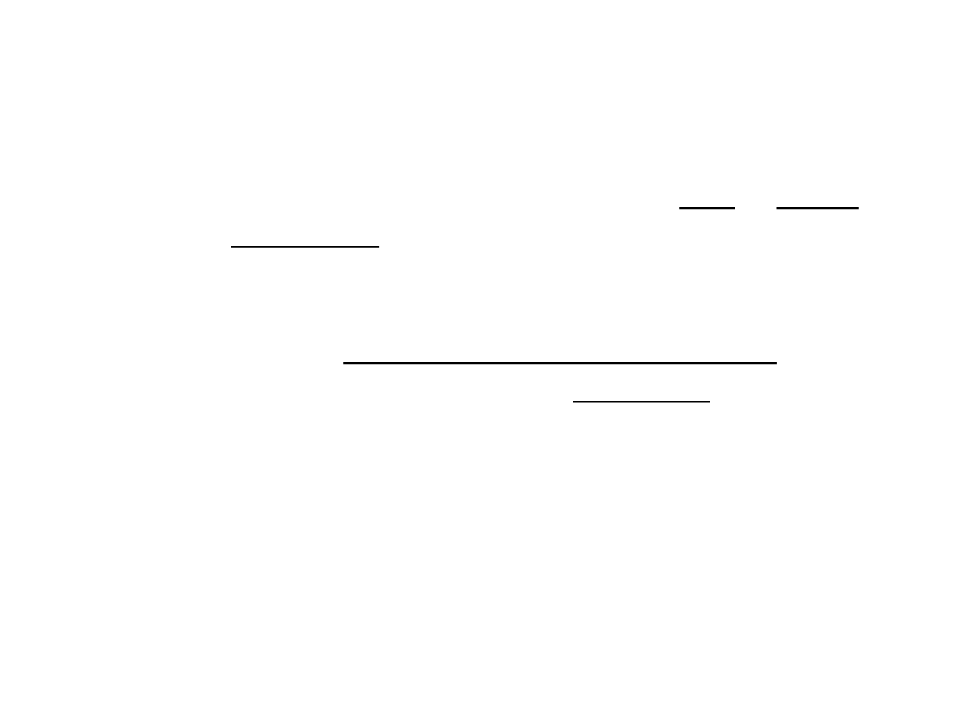Hash Table
A hash table is a data structure that can map keys to values.
It uses a hash function to compute an index where the desired value
can be found.
A hash table offers very fast insertions and searching methods.
The performance of hash tables is often close to O(1) in most of its
operations, but it depends on a number of factors such as the overall
collisions.
This performance comes from the fact that hash tables are based on
the array data structure.
When the array index of an object is known, the position is nothing more than a
table lookup using the array index.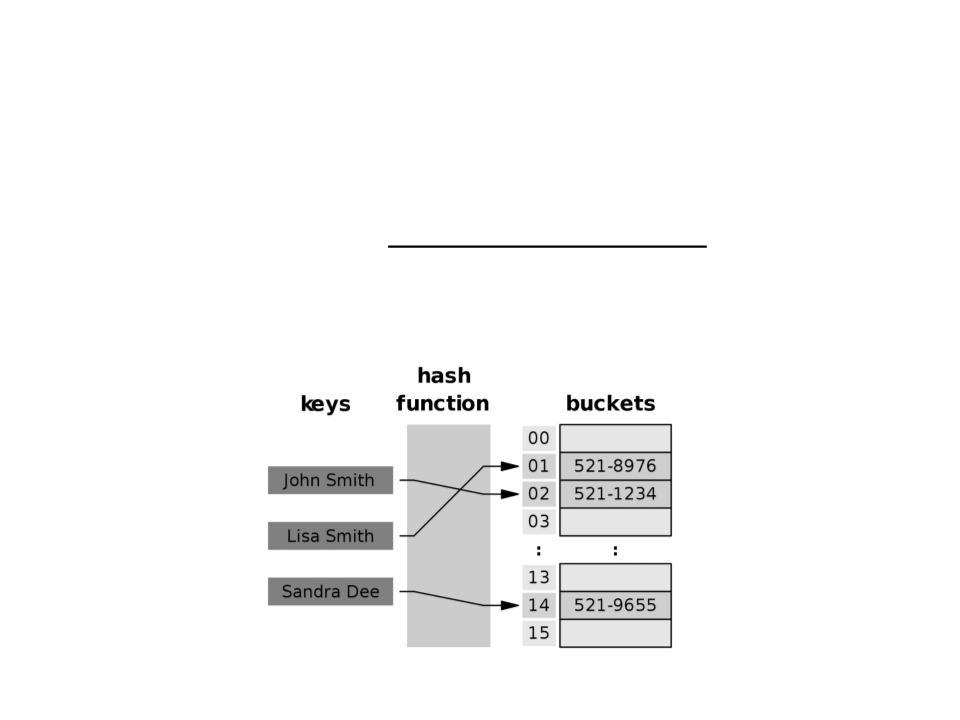Hash Function
A hash function is an algorithm that takes a value and
compresses it into the range of the hash table’s array.
This value is known as the key, and it can be anything from a string to a
number.Hash Function
Expected properties:
The hash value is fully determined by the data being hashed.
The hash function uses all the input data.
“Uniformly" distributes the data across the entire set of hash values.
Generates very different hash values for similar inputs.
Example of hash function for string keys:
private int HashFunction(string key){
long index = 7;
int asciiCode = 0;
for (int i = 0; i < key.Length; i++){
asciiCode = (int)key[i] * i;
index = index * 31 + asciiCode;
}
return (int)(index % tableSize);
}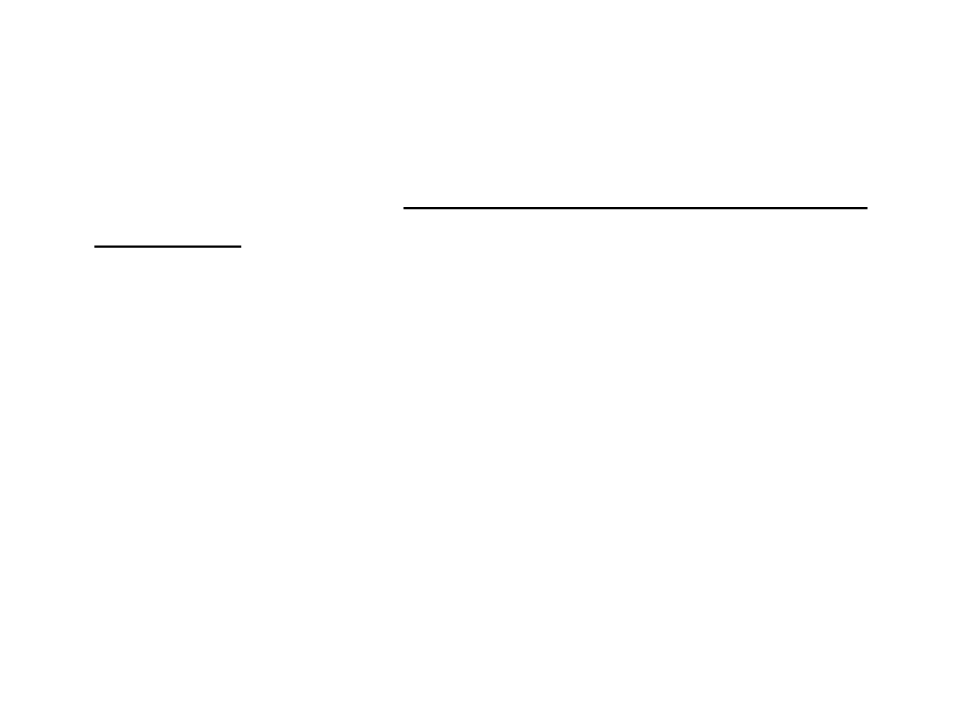Collisions
A collision occurs when more than one object hashes to the
same index.
Using a poor hash function can lead to more collisions.
Even with good hash functions, there are still chances of collisions.
What to do when two or more keys are hashed to same
index?
One solution is to have a linked list at every index.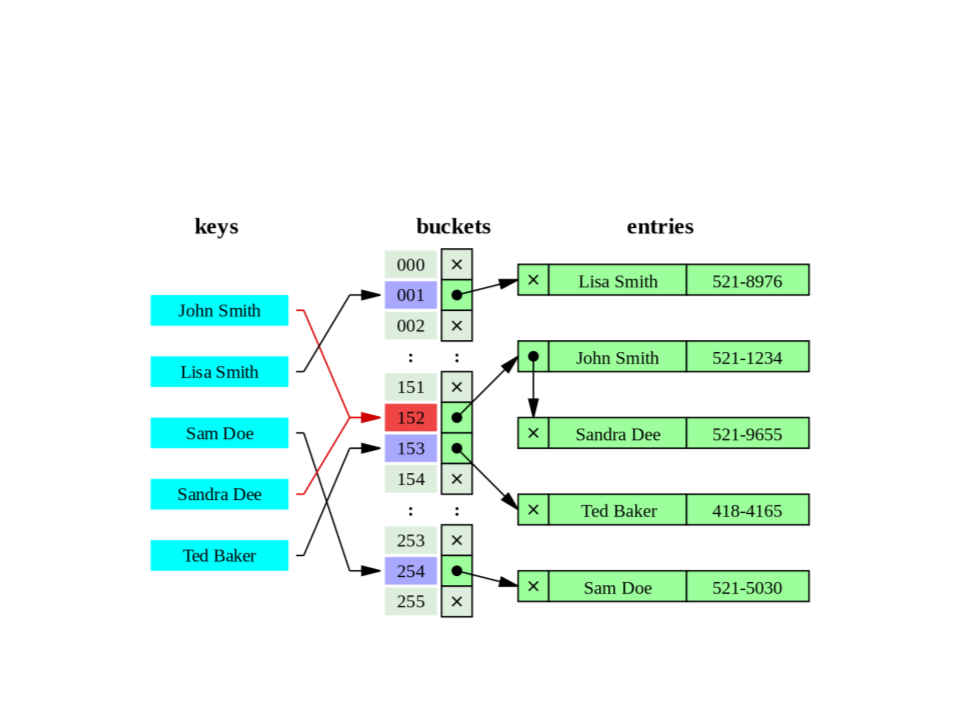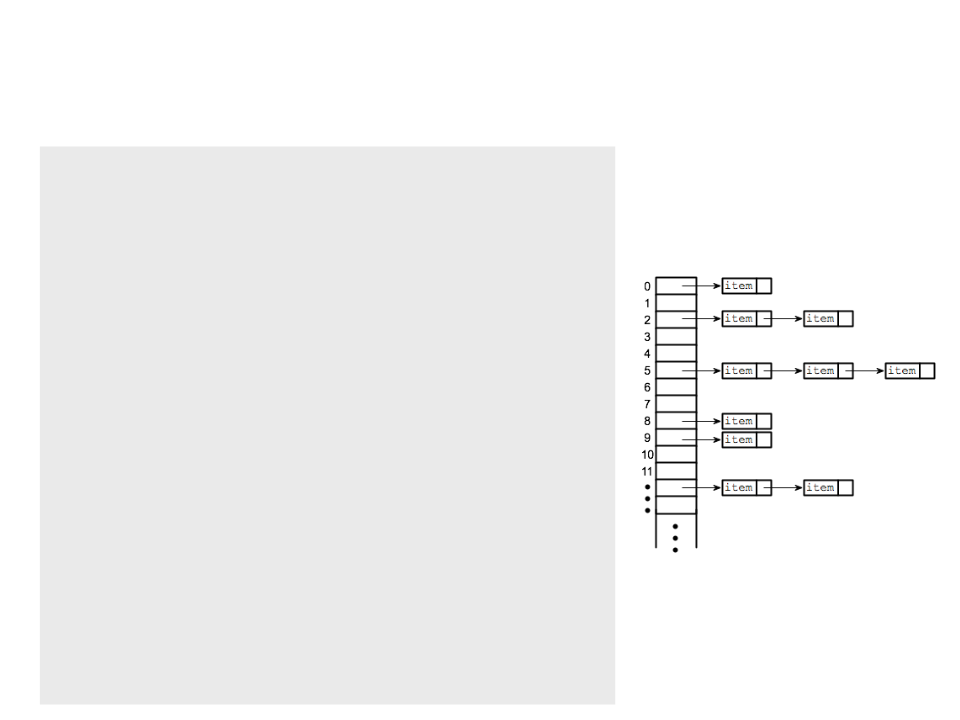Hash Table Structure
public class HashtableNode{
public string Key { get; set; }
public object Value { get; set; }
public HashtableNode Next { get; set; }
public HashtableNode(string key, object value){
Key = key;
Value = value;
Next = null;
}
}
public class Hashtable{
private HashtableNode[] buckets;
private int tableSize;
public Hashtable(int maxTableSize){
tableSize = maxTableSize;
buckets = new HashtableNode[tableSize];
}
.
..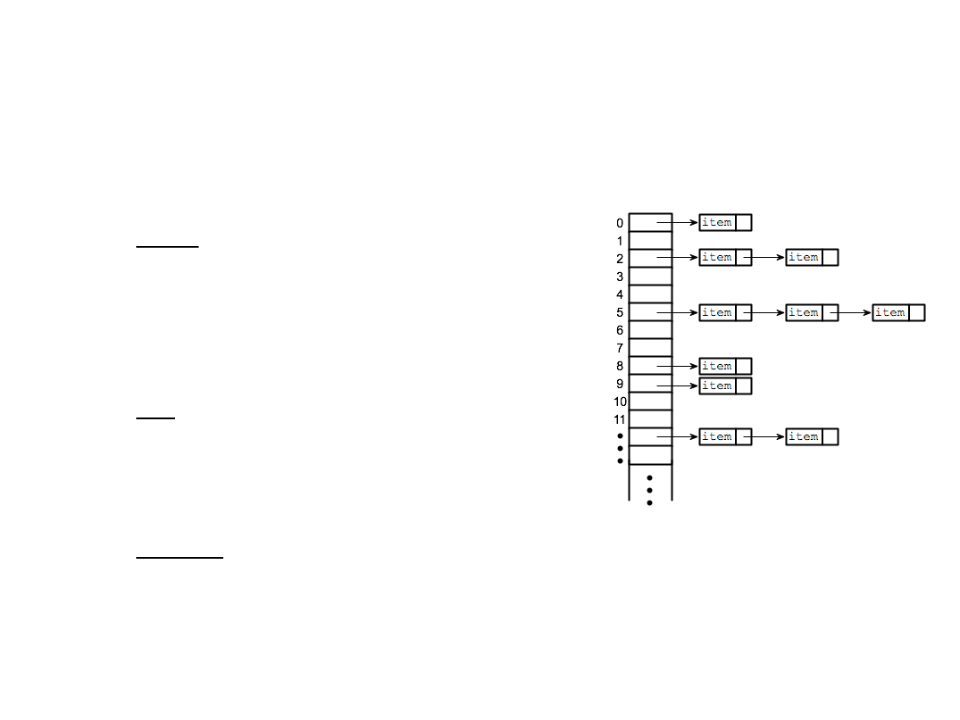Hash Table
Basic operations:
Insert: inserts an element into the
hash table according to a key-value
pair.
Get: returns the value associated with
a given key.
Return null if the key does not exist.
Remove: removes an element from the
hash table according to a given key.Hash Table Insert
public void Insert(string key, object value){
int hashIndex = HashFunction(key);
HashtableNode node = buckets[hashIndex];
if (node == null)
buckets[hashIndex] = new HashtableNode(key, value);
else{
if (node.Key == key)
node.Value = value;
else{
while (node.Next != null){
node = node.Next;
if (node.Key == key)
node.Value = value;
}
HashtableNode newNode = new HashtableNode(key, value);
node.Next = newNode;
}
}
}Hash Table Get
Returns the value associated with a given key.
Return null if the key does not exist.
public object GetValue(string key)
{
int hashIndex = HashFunction(key);
HashtableNode node = buckets[hashIndex];
while (node != null)
{
if (node.Key == key)
{
return node.Value;
}
node = node.Next;
}
return null;
}Hash Table Remove
public bool Remove(string key){
int hashIndex = HashFunction(key);
HashtableNode node = buckets[hashIndex];
if (node == null)
return false;
if (node.Key == key)
{
buckets[hashIndex] = node.Next;
return true;
}
HashtableNode prev = node;
while (node != null){
if (node.Key == key){
prev.Next = node.Next;
return true;
}
prev = node;
node = node.Next;
}
return false;
}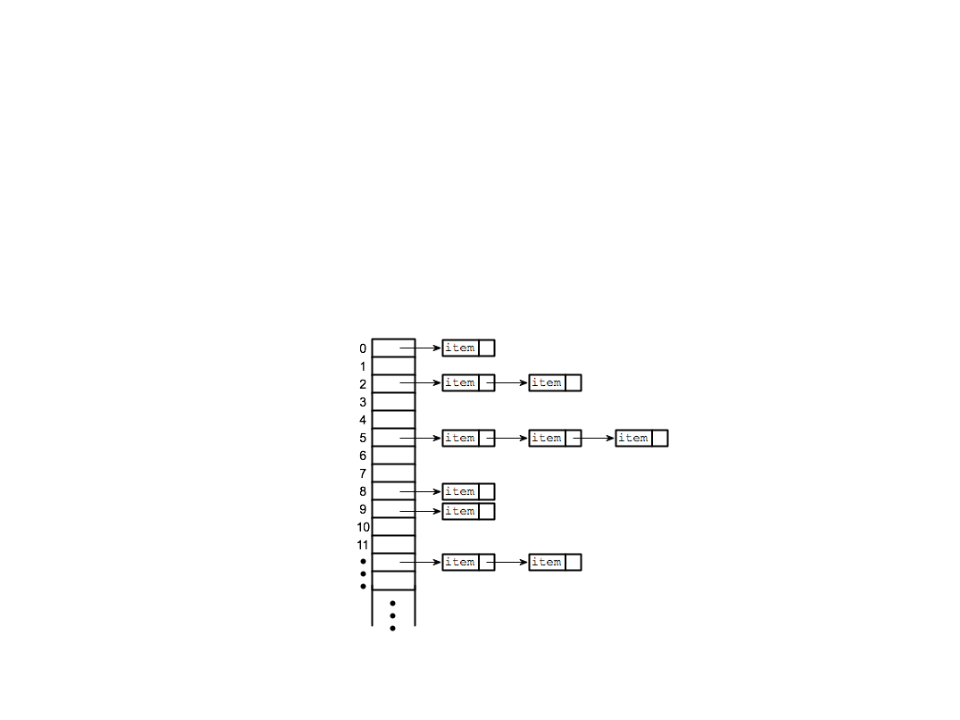Applications in Game Programming
Store and access dynamic set of objects that can be indexed
by a key: list of resources, list of enemies, plans of actions,
game states, layers, etc.Assignment Hash Table
1
) Continue the implementation of the HashTable class by
implementing the following operations:
a. bool ContainsKey(string key): determines whether the hash table
contains a specific key.
b. bool ContainsValue(object value): determines whether the hash
table contains a specific value.
c. int Count(): returns the number of elements that are in the hash
table.